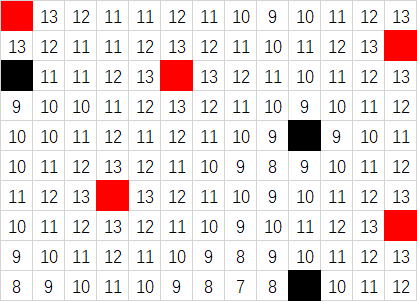HPU第二次积分赛

# A. 再战斐波那契

$$Fibonacci[i]== \begin{cases} i& \text{x<=1}\ Fibonacci[i−1]+Fibonacci[i−2]& \text{x>1} \end{cases}$$

Input

3
1 2
2 3
3 4

Output

1
1
1

#include<iostream>
#include<algorithm>
using namespace std;
typedef long long ll;
ll a;
int main()
{
ios::sync_with_stdio(false);
a=0;a=1;
for(int i=2;i<94;i++)
a[i]=a[i-1]+a[i-2];
ll t,x,y;
while(cin>>t)
{
while(t--)
{
cin>>x>>y;
cout<<a[__gcd(x,y)]<<endl;
}
}
return 0;
}

# B. 恐怖的怪物

此规律在坐标轴的3个方向上（东西、南北、上下）均成立。换句话来说，对角线方向的光照衰减依照“曼哈顿距离”（两个点在坐标系上的绝对轴距总和）计算。这意味着，假如地上插着一支火把（光照等级14），则在水平面上与火把相邻的4个方向的方格上光照等级均为13，而在水平面上与火把对角的4个方格上光照等级均为12（譬如，西北方格的光照等级为14-向西1级-向北1级）。
上述这种衰减特性会在光源周围产生菱形的照明。该效果会在光源周围的光源扩散呈钻石状。如果被不透明方块阻挡，光照也可以沿着复杂而弯曲的路径扩散。秘密基地为N∗M的空间，不考虑高度，初始地面光照强度为0。为了不生成恐怖的怪物，Dicer布置了一些光源，但他不知道是否仍会生成怪物，现在请你帮助Dicer判断。

Input

2
2 3
0Y0
00#
3 4
R00#
00R0
0R00

Output
No
Yes
Input

2
1 5
0Y0R0
2 4
Y#0R
0000

Output
Yes
No
Input

1
5 4
Y0F0
0000
0000
0000
0000

Output
No

#include<iostream>
#include<stdio.h>
#include<algorithm>
#include<string.h>
#include<string>
#include<queue>
#include<stack>
#include<set>
#include<map>
#include<vector>
#include<math.h>
using namespace std;
typedef long long ll;
typedef unsigned long long  ull;

const int maxn=1e5+10;
const int mod=1e9+7;

char mmp;//存图
int mp;//存光照强度
bool flag;//作为标记
struct pe{
int x,y;
int s;
bool friend operator < (pe x,pe y)//规定一下排列顺序
{
return x.s<y.s ;
}
}cc,c;
int t,n,m;
int dir={1,0,0,1,-1,0,0,-1};

priority_queue<pe>q;

bool bfs()
{
c=q.top() ;

while(!q.empty() )
{
c=q.top() ;q.pop() ;
if(mp[c.x][c.y]==8) break;
for(int i=0;i<4;i++)
{
int x=c.x+dir[i] ;int y=c.y+dir[i] ;
//注意这里只有mmp[x][y]=='0'；才能放入队列；
if(x>=0&&x<n&&y>=0&&y<m&&!flag[x][y]&&mmp[x][y]=='0'&&mp[x][y]<c.s)
{
flag[x][y]=1;
mp[x][y]=c.s -1;
cc.x =x;cc.y =y;cc.s =c.s -1;
q.push(cc);
}
}
}
for(int i=0;i<n;i++)
{
for(int j=0;j<m;j++)
{
if(mmp[i][j]=='0'&&mp[i][j]<=7)//判断是不是满足条件
return 0;
}
}
return 1;
}

int main()
{
ios::sync_with_stdio(false);
while(cin>>t)
{
while(t--)
{
cin>>n>>m;
memset(mp,0,sizeof mp);
memset(flag,0,sizeof flag);
for(int i=0;i<n;i++)
{
cin>>mmp[i];
for(int j=0;j<m;j++)//存图并且提前判断一下光照强度标记光源和记录强度
{
if(mmp[i][j]=='Y') {
mp[i][j]=15;//flag[i][j]=1;
c.x=i;c.y=j;c.s =15;
q.push(c);
}
else if(mmp[i][j]=='H'){
mp[i][j]=14;    //flag[i][j]=1;
c.x=i;c.y=j;c.s =14;
q.push(c);
}
else if(mmp[i][j]=='R')
{
mp[i][j]=7;//flag[i][j]=1;
c.x=i;c.y=j;c.s =7;
q.push(c);
}
else if(mmp[i][j]=='F')
{
mp[i][j]=12;//flag[i][j]=1;
c.x=i;c.y=j;c.s =12;
q.push(c);
}

}
}
if(bfs()) cout<<"No"<<endl;
else cout<<"Yes"<<endl;
}
}
return 0;
}

# C. 连连看

众所周知，《连连看》是一个老少皆宜的游戏。
《连连看》是由黄兴武创作的一款PC端益智类游戏，只要将相同的两张牌用三根以内的线段连在一起就可以消除，规则简单容易上手。

现在呢，Boctorio学长突然想玩连连看了，但不是单纯的玩游戏，他想自己出一局连连看。

Input

3
2 2
1 2
2 1
3 4
1 6 2 3
4 5 3 1
4 2 6 5
4 4
1 2 3 6
8 4 7 8
5 6 5 7
1 2 3 4

Output
No
No
Yes

emmmmm这个题之前写过一个简单的但是现在还是不会以后再补吧…

# D. Points in rectangle

在二维平面中有一个矩形，它的四个坐标点分别为(0,a),(a,0),(n,n−a),(n−a,n)。你现在有m个点，现在你想知道有多少个点是在这个矩形内的(边上的也算)。

Input

6 1
5
1 2
1 3
2 3
3 4
4 5

Output

4
4 5
3 4
2 3
1 2

#include<queue>
#include<string>
#include<queue>
#include<stack>
#include<string.h>
#include<set>
#include<map>
#include<algorithm>
#include<iostream>
#include<stdio.h>

using namespace std;
typedef pair<int,int> pa;
typedef long long ll;

const int maxn=1e5+10;
const int mod=1e9+7;
struct pe{
int x,y;
}S;
bool cmp(pe x,pe y){
if(x.x !=y.x ) return x.x >y.x ;
else return x.y >y.y ;
}
int n,m,k;
int x,y;
int main()
{
ios::sync_with_stdio(false);
cin>>n>>m;
cin>>k;
int bb=2*n-m;
int t=0;
for(int i=0;i<k;i++)
{
cin>>x>>y;
int w=y-x-m,q=y-x+m;
int r=y+x-m,s=y+x-bb;
if((q>=0&&w<=0)&&(r>=0&&s<=0))
{
S[t].x =x;
S[t++].y=y;
}
}
if(!t) cout<<-1<<endl;
else
{
sort(S,S+t,cmp);
cout<<t<<endl;
for(int i=0;i<t;i++)
{
cout<<S[i].x <<" "<<S[i].y<<endl;
}
}
return 0;
}

# E. Numbers of interval

Input

3 5
2 3 5

Output
4

#include<queue>
#include<string>
#include<queue>
#include<stack>
#include<string.h>
#include<set>
#include<map>
#include<algorithm>
#include<iostream>
#include<stdio.h>

using namespace std;
typedef pair<int,int> pa;
typedef long long ll;
const int maxn=1e6+1000;
const int mod=1e9+7;
int a[maxn];

int main()
{
ios::sync_with_stdio(false);
ll n,m;
cin>>n>>m;
memset(a,0,sizeof a);
ll sum=0;
ll ast=0;//初始化，记录有几种方案
ll k=0;
for(ll i=0;i<n;i++)
{
cin>>a[i];
sum+=a[i];//前几项的累加
if(sum>=m)//如果大于m就开始减；
{
ast+=n-i;
for(;k<=i;)//最多减到当前位置；
{
sum-=a[k++];
if(sum>=m) ast+=n-i;//如果依旧满足条件那么就一直加；
else break;
}
}
}
cout<<ast<<endl;
return 0;
}

# I. Same String

有两个只由小写字母组成的长度为n的字符串s1,s2和m组字母对应关系，每一组关系由两个字母c1和c2组成，代表c1可以直接变成c2,你需要判断s1是否可以通过这m组关系转换为s2。

Input

6
aabbcc
4
a c
c a
a d
b c

Output
YES

#include<queue>
#include<string>
#include<queue>
#include<vector>
#include<string.h>
#include<set>
#include<map>
#include<algorithm>

#include<stdio.h>

using namespace std;
typedef pair<int,int> pa;
typedef long long ll;

const int maxn=1e5+10;
const int mod=1e9+7;

char a,aa;
vector<int> v;
bool flag;
bool bfs(int x,int y)
{//每次都要初始化！！！
memset(flag,0,sizeof(flag));
queue<int>q;
q.push(x);
flag[x]=1;
while(!q.empty() )
{
int xx=q.front() ;q.pop() ;
if(xx==y){
return 0;
}
else{
for(int i=0;i<v[xx].size() ;i++)
{
int qq=v[xx][i];
if(!flag[qq])
{
q.push(qq);
flag[qq]=1;
}
}
}
}
return 1;
}

int main()
{
//    ios::sync_with_stdio(false);
int n,m;
char x,y;
scanf("%d",&n);
scanf("%s",a);
scanf("%s",aa);
scanf("%d",&m);
getchar();
for(int i=0;i<m;i++)
{
scanf("%c %c",&x,&y);
getchar();
int A=x-'a';int B=y-'a';
v[A].push_back(B); //存入邻接表
}
int fa=0;
for(int i=0;i<n;i++)
{
if(a[i]!=aa[i])
{
if(bfs(a[i]-'a',aa[i]-'a')){//每次判断
fa=1;
break;
}
}
}
if(fa) puts("NO");
else puts("YES");
return 0;
}
//这里附加一份华佬的代码，用了另一个算法，还是比较巧的，华佬真强！！！
#include<bits/stdc++.h>
using namespace std;
typedef long long ll;
int n,m;
string s1,s2;
char c,d;
int ma;
int main(){
ios::sync_with_stdio(0);
cin >> n >> s1 >> s2 >> m;
for(int i = 0; i < m; i++){
cin >> c >> d;
ma[c][d] = 1;
}
for(int i = 'a'; i <= 'z'; i++){
for(int j = 'a'; j <= 'z'; j++){
if(ma[j][i]){
for(int k = 'a'; k <= 'z'; k++){
ma[j][k] = ma[j][k] || ma[i][k];
}
}
}
}
for(int i = 0; i < n; i++){
if(s1[i] != s2[i]){
if(!ma[s1[i]][s2[i]]) {
cout << "NO\n";
return 0;
}
}
}
cout <<"YES\n";

return 0;
}

上一篇POJ-2585-Window Pains
POJ-2585-Window Pains Window Pains Time Limit: 1000MS Memory Limit: 65536K Total Submissions: 2915 Accepted: 1
2019-07-28树的直径

2019-07-25
目录